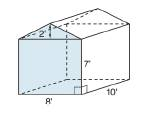Chapter 9.1, Problem 29EElementary Geometry For College St...

7th Edition
Alexander + 2 others
ISBN: 9781337614085

Solutions

Chapter
SectionElementary Geometry For College St...

7th Edition
Alexander + 2 others
ISBN: 9781337614085
Textbook Problem

A storage shed is in the shape of a pentagonal prism. The front represents one of its two pentagonal bases. What is the storage capacity (volume) of its interior?To determine

To find:

The storage capacity (volume) of a storage shed in the shape of a pentagonal prism.

Explanation

The volume of any solid is the region of space occupied by it and in general the volume of a solid is given by the product of its base area and altitude.

For a regular solid prism with base area B and altitude h, the volume is given by the formula

V=Bh.

Calculation:

Given,

A pentagonal prism with an irregular pentagonal base.

The pentagonal base has two sections, one a rectangular section of dimension 8 in.  × 7 in. and a triangular section of base 8 in and height 2 in.

The height of the shed is 10 in.

The capacity (volume) of the storage shed (pentagonal prism) with base area B and height ‘h’ is given by the formula

V=Bh

Let us calculate the base area ‘B’ of the pentagonal prism.

Since, the base is not a regular pentagonal, its area is given by

B= Area of the rectangular part of the base + Area of the triangular part of the base

Still sussing out bartleby?

Check out a sample textbook solution.

See a sample solution

The Solution to Your Study Problems

Bartleby provides explanations to thousands of textbook problems written by our experts, many with advanced degrees!

Get Started

Find more solutions based on key concepts